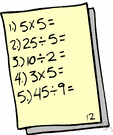# arithmetic

(redirected from Arithmatic)
Also found in: Thesaurus, Encyclopedia.

## a·rith·me·tic

(ə-rĭth′mĭ-tĭk)
n.
The mathematics of integers, rational numbers, real numbers, or complex numbers under addition, subtraction, multiplication, and division.
adj. ar·ith·met·ic (ăr′ĭth-mĕt′ĭk) also ar′ith·met′i·cal (ăr′ĭth-mĕt′ĭ-kəl)
1. Of or relating to arithmetic.
2. Changing according to an arithmetic progression: The increase in the food supply is arithmetic.

[Middle English arsmetike, from Old French arismetique, from Medieval Latin arismetica, alteration of Latin arithmētica, from Greek arithmētikē (tekhnē), (art) of counting, feminine of arithmētikos, from arithmein, to count, from arithmos, number; see ar- in Indo-European roots.]

a·rith′me·ti′cian (-tĭsh′ən) n.

## arithmetic

(əˈrɪθmətɪk)
n
1. (Mathematics) the branch of mathematics concerned with numerical calculations, such as addition, subtraction, multiplication, and division
2. (Mathematics) one or more calculations involving numerical operations
3. (Mathematics) knowledge of or skill in using arithmetic: his arithmetic is good.
(Mathematics) of, relating to, or using arithmetic
[C13: from Latin arithmētica, from Greek arithmētikē, from arithmein to count, from arithmos number]
aˌrithmeˈtician n

## a•rith•me•tic

(n. əˈrɪθ mə tɪk; adj. ˌær ɪθˈmɛt ɪk)

n.
1. the method or process of computation with figures: the most elementary branch of mathematics.
2. the theory of numbers; the study of the divisibility of whole numbers, the remainders after division, etc.
3. a treatise on arithmetic.
4. Also, ar`ith•met′i•cal. of, pertaining to, or in accordance with the rules of arithmetic.
ar•ith•met•ic
[1200–50; < Old French arismetique < Medieval Latin arismētica, Latin arithmētica < Greek arithmētikḗ (téchnē) (art, skill) of numbers, feminine of arithmētikós <arithmēt(ós) number]

## a·rith·me·tic

(ə-rĭth′mĭ-tĭk)
1. The mathematical study of numbers and their properties under the operations of addition, subtraction, multiplication, and division.
2. Calculation using these operations.
ThesaurusAntonymsRelated WordsSynonymsLegend:
 Noun 1arithmetic - the branch of pure mathematics dealing with the theory of numerical calculationsmath, mathematics, maths - a science (or group of related sciences) dealing with the logic of quantity and shape and arrangementpure mathematics - the branches of mathematics that study and develop the principles of mathematics for their own sake rather than for their immediate usefulnessalgorism - computation with Arabic figuresmiscalculate, misestimate - calculate incorrectly; "I miscalculated the number of guests at the wedding"recalculate - calculate anew; "The costs had to be recalculated"average out, average - compute the average ofsquare - raise to the second powercube - raise to the third powerfactor, factor in, factor out - resolve into factors; "a quantum computer can factor the number 15"add together, add - make an addition by combining numbers; "Add 27 and 49, please!"foot up, foot - add a column of numbersdeduct, subtract, take off - make a subtraction; "subtract this amount from my paycheck"multiply - combine by multiplication; "multiply 10 by 15"raise - multiply (a number) by itself a specified number of times: 8 is 2 raised to the power 3fraction, divide - perform a division; "Can you divide 49 by seven?"halve - divide by two; divide into halves; "Halve the cake"quarter - divide by four; divide into quartersmake - add up to; "four and four make eight"contain - be divisible by; "24 contains 6" Adj. 1arithmetic - relating to or involving arithmetic; "arithmetical computations"arithmetical

## arithmetic

noun
Arithmetic calculations
computation, figure (used in plural), number (used in plural).
Translations
عِلْم الحِساب
aritmetikaaritmetický
aritmetik
arithmétiquecalculd'arithmétiquede l’arithmétiquemoyenne
reikningur, talnafræîi

aritmetikaaritmetinis
aritmētika
aritmeticarekenkunde
aritmetika
aritmetika
aritmetikaritmetisk

## arithmetic

A. [əˈrɪθmətɪk] N
arithmetic progression
C. [ˌærɪθˈmetɪk] CPD arithmetic mean N

## arithmetic

[əˈrɪθmɪtɪk] n
(= school subject) →
(= calculations) → calculs mpl
He got his arithmetic wrong → Il s'est trompé dans ses calculs.
if my arithmetic is right ... → si mes calculs sont bons ...
the arithmetic (of particular situation)l'arithmétique

## arithmetic

nRechnen nt; (= calculation)Rechnung f; could you check my arithmetic?kannst du mal gucken, ob ich richtig gerechnet habe?; your arithmetic is wrongdu hast dich verrechnet; arithmetic bookRechenfibel for -buch nt; (= exercise book)Rechenheft nt

## arithmetic

[n əˈrɪθmətɪk; adj ˌærɪθˈmɛtɪk]
1. naritmetica
mental arithmetic → calcolo mentale
arithmetic progression →

## arithmetic

(əˈriθmətik) noun
the art of counting by numbers.